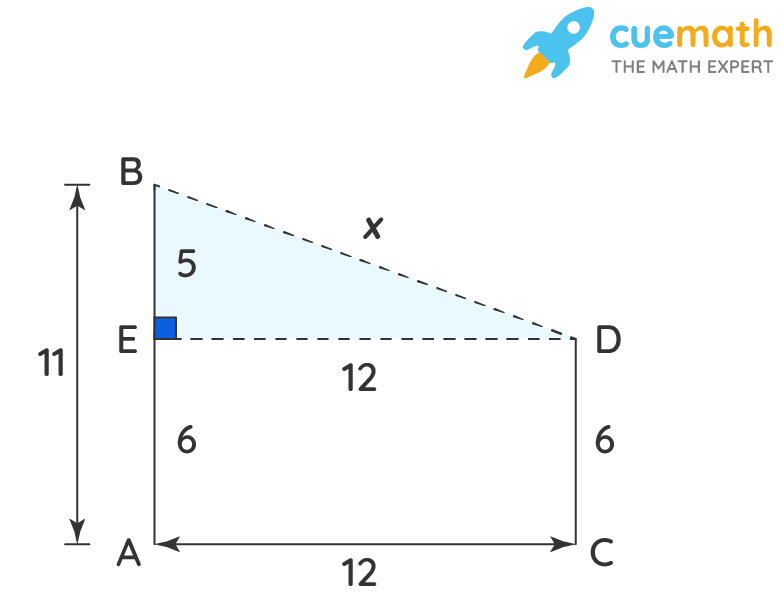# Two Poles of Height 6m and 11m Stands on a Plane Ground, Find the Distance Between Their Tops.

## Question: Two poles of height 6m and 11m stands on a plane ground, if the distance between their feet is 12m. Find the distance between their tops.

The Pythagoras theorem states that if a triangle is right-angled (90 degrees), then the square of the hypotenuse is equal to the sum of the squares of the other two sides.

## Answer: Two poles of height 6m and 11m stands on a plane ground, where distance between their feet is 12m, the distance between the tops of the two poles is 13 m.

The Pythagoras theorem works only for right-angled triangles. When any two values are known, we can apply the theorem and calculate the third side.

## Explanation:

Let's draw the diagram of the two poles AB and CD as shown:Let, BD be the distance between the two poles

Assume, BD = x

As △BED is a right-angled triangle right angled at E, therefore 'x' is the hypotenuse.

Now apply the Pythagoras theorem on △BED ,

Hypotenuse2 = Perpendicular2 + Base2

=> BD2 = ED2 + BE2

=> x2 = 122 + 52

=> x2 = 144 + 25

=> x2 = 169

=> x = 13 m